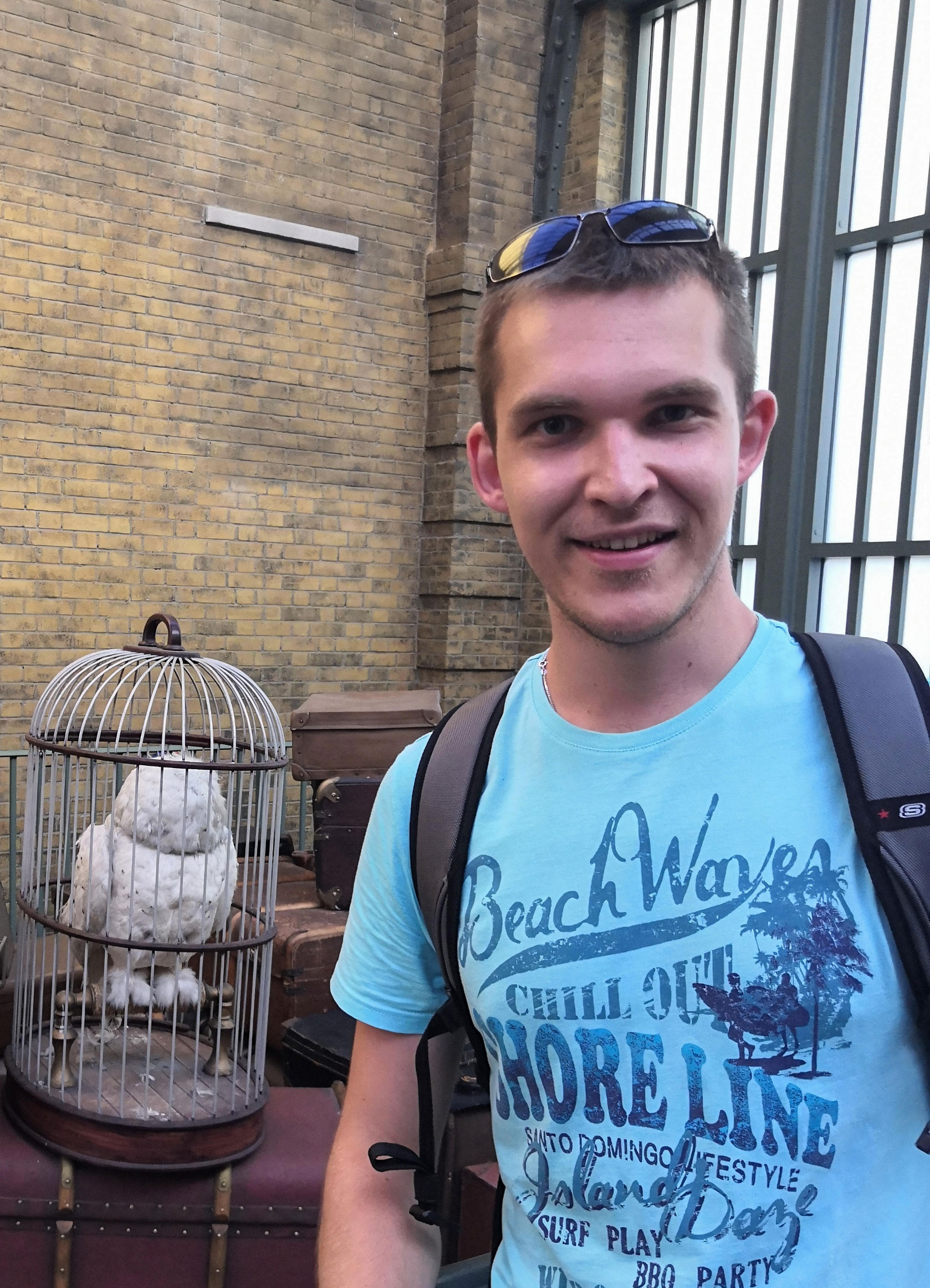﻿ Laboratory site
En / Ru## Valiullin Albert Radikovich

→ Graduate of the Faculty of Mechanics and Mathematics at Lomonosov Moscow State University

E-mail:

Keywords: greedy algorithms, approximation theory, frames.

### Main result:

In greedy expansions with prescribed coefficients in Hilbert spaces, we could obtain sharp sufficient conditions for the convergence of the greedy expansion to the expanded element. Specifically, if the sequence of coefficients $$\{c_n\}_{n = 1}^{\infty}$$ satisfies the conditions $$c_n = o\left(\frac{1}{\sqrt{n}}\right)$$ and $$\sum \limits_{n = 1}^{\infty}c_n = \infty$$, then convergence is guaranteed. On the other hand, convergence can be violated for $$c_n \sim \frac{1}{\sqrt{n}}$$.

### Publications:

2022

→ Al.R. Valiullin, Ar.R. Valiullin, A.P. Solodov, "Sharp sufficient condition for the convergence of greedy expansions with errors in coefficient computation", Demonstratio Mathematica, 55:1 (2022), 254–264, DOI.

2021

→ Al. R. Valiullin, Ar. R. Valiullin, V. V. Galatenko, "Frames as continuous redundant codes", Vestnik Moskov. Univ. Ser. 1. Mat. Mekh., 2 (2021), 39–43, link.

→ Ar. R. Valiullin, Al. R. Valiullin, "Sharp conditions for the convergence of greedy expansions with prescribed coefficients", Open Mathematics, 19:1 (2021), 1–10, DOI, PDF.

2018

→ Ar. R. Valiullin, Al. R. Valiullin, V. V. Galatenko, "Greedy expansions with prescribed coefficients in Hilbert spaces", Int. Jour. of Math. and Math. Sc. DOI, PDF.